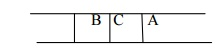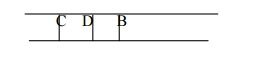Home | | Artificial Intelligence | Uninformed Search

# Uninformed Search

Breadth First Search (BFS), Depth First Search (DFS), Brute Force or Blind Search, Greedy Search

UNINFORMED SEARCH

Breadth first search is a general technique of traversing a graph. Breadth first search may use more memory but will always find the shortest path first. In this type of search the state space is represented in form of a tree. The solution is obtained by traversing through the tree. The nodes of the tree represent the start value or starting state, various intermediate states and the final state. In this search a queue data structure is used and it is level by level traversal. Breadth first search expands nodes in order of their distance from the root. It is a path finding algorithm that is capable of always finding the solution if one exists. The solution which is found is always the optional solution. This task is completed in a very memory intensive manner. Each node in the search tree is expanded in a breadth wise at each level.

Concept:

Step 1: Traverse the root node

Step 2: Traverse all neighbours of root node.

Step 3: Traverse all neighbours of neighbours of the root node.

Step 4: This process will continue until we are getting the goal node.

Algorithm:

Step 1: Place the root node inside the queue.

Step 2: If the queue is empty then stops and return failure.

Step 3: If the FRONT node of the queue is a goal node then stop and return success.

Step 4: Remove the FRONT node from the queue. Process it and find all its neighbours that are in ready state then place them inside the queue in any order.

Step 5: Go to Step 3.

Step 6: Exit.

Implementation:

Let us implement the above algorithm of BFS by taking the following suitable example.Consider the graph in which let us take A as the starting node and F as the goal node (*)

Step 1:

Place the root node inside the queue i.e. AStep 2:

Now the queue is not empty and also the FRONT node i.e. A is not our goal node. So move to step 3.

Step 3:

So remove the FRONT node from the queue i.e. A and find the neighbour of A i.e. B and CStep 4:

Now b is the FRONT node of the queue .So process B and finds the neighbours of B i.e. D.Step 5:

Now find out the neighbours of C i.e. EIn this procedure at any way it will find the goal.

It does not follow a single unfruitful path for a long time. It finds the minimal solution in case of multiple paths.

BFS consumes large memory space. Its time complexity is more.

It has long pathways, when all paths to a destination are on approximately the same search depth.

Depth First Search (DFS)

DFS is also an important type of uniform search. DFS visits all the vertices in the graph. This type of algorithm always chooses to go deeper into the graph. After DFS visited all the reachable vertices from a particular sources vertices it chooses one of the remaining undiscovered vertices and continues the search. DFS reminds the space limitation of breath first search by always generating next a child of the deepest unexpanded nodded. The data structure stack or last in first out (LIFO) is used for DFS. One interesting property of DFS is that, the discover and finish time of each vertex from a parenthesis structure. If we use one open parenthesis when a vertex is finished then the result is properly nested set of parenthesis.

Concept:

Step 1: Traverse the root node.

Step 2: Traverse any neighbour of the root node.

Step 3: Traverse any neighbour of neighbour of the root node.

Step 4: This process will continue until we are getting the goal node.

Algorithm:

Step 1: PUSH the starting node into the stack.

Step 2: If the stack is empty then stop and return failure.

Step 3: If the top node of the stack is the goal node, then stop and return success.

Step 4: Else POP the top node from the stack and process it. Find all its neighbours that are in ready state and PUSH them into the stack in any order.

Step 5: Go to step 3.

Step 6: Exit.

Implementation:

Let us take an example for implementing the above DFS algorithm.Consider A as the root node and L as the goal node in the graph figure

Step 1: PUSH the starting node into the stack i.e.Also you can traverse the graph starting from the root A and then insert in the order C and B into the stack. Check your answer.

DFSconsumes very less memory space.

It will reach at the goal node in a less time period than BFS if it traverses in a right path.

It may find a solution without examining much of search because we may get the desired solution in the very first go.

It is possible that may states keep reoccurring. There is no guarantee of finding the goal node.

Sometimes the states may also enter into infinite loops.

Brute Force or Blind Search

Brute force or blind search is a uniformed exploration of the search space and it does not explicitly take into account either planning efficiency or execution efficiency. Blind search is also called uniform search. It is the search which has no information about its domain. The only thing that a blind search can do is to differentiate between a non goal state and a goal state. These methods do not need domain knowledge but they are less efficient in result. Uniform strategies donâ€™t use any information about how a close a node might be to a goal. They differ in the order that the nodes are expanded. The most important brute force techniques are breadth first search, depth first search, uniform search and bidirectional search. All brute force techniques must take ( b0 time and use o (d) space. This technique is not as efficient as compared to other algorithms.

Difference between BFS and DFS

BFS

It uses the data structure queue.

BFS is complete because it finds the solution if one exists.

BFS takes more space i.e. equivalent to o (b0) where b is the maximum breath exist in a search tree and d is the maximum depth exit in a search tree.

In case of several goals, it finds the best one.

DFS

It uses the data structure stack.

It is not complete because it may take infinite loop to reach at the goal node. The space complexity is O (d).

In case of several goals, it will terminate the solution in any order.

Greedy Search

This algorithm uses an approach which is quite similar to the best first search algorithm. It is a simple best first search which reduces the estimated cost of reach the goal. Basically it takes the closest node that appears to be closest to the goal. This search starts with the initial matrix and makes very single possible changes then looks at the change it made to the score. This search then applies the change till the greatest improvement. The search continues until no further improvement can be made. The greedy search never makes never makes a lateral move .It uses minimal estimated cost h (n) to the goal state as measure which decreases the search time but the algorithm is neither complete nor optimal. The main advantage of this search is that it is simple and finds solution quickly. The disadvantages are that it is not optimal, susceptible to false start.Study Material, Lecturing Notes, Assignment, Reference, Wiki description explanation, brief detail
Artificial Intelligence : Uninformed Search |